Updating search results...

# 131 Results

View
Selected filters:
• NSDLUnrestricted Use
CC BY
Rating
0,0 stars

This task examines the ways in which the plane can be covered by regular polygons in a very strict arrangement called a regular tessellation. These tessellations are studied here using algebra, which enters the picture via the formula for the measure of the interior angles of a regular polygon (which should therefore be introduced or reviewed before beginning the task). The goal of the task is to use algebra in order to understand which tessellations of the plane with regular polygons are possible.

نوع المادة:
Activity/Lab
Provider:
Illustrative Mathematics
Provider Set:
Illustrative Mathematics
المؤلف:
Illustrative Mathematics
01/21/2013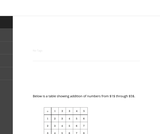Unrestricted Use
CC BY
Rating
3,0 stars

The purpose of this task is to study some patterns in a small addition table. Each pattern identified persists for a larger table and if more time is available for this activity students should be encouraged to explore these patterns in larger tables.

نوع المادة:
Activity/Lab
Provider:
Illustrative Mathematics
Provider Set:
Illustrative Mathematics
المؤلف:
Illustrative Mathematics
08/15/2012Conditional Remix & Share Permitted
CC BY-NC-SA
Rating
0,0 stars

In this activity, learners use a hand-made protractor to measure angles they find in playground equipment. Learners will observe that angle measurements do not change with distance, because they are distance invariant, or constant. Note: The "Pocket Protractor" activity should be done ahead as a separate activity (see related resource), but a standard protractor can be used as a substitute.

نوع المادة:
Activity/Lab
Provider:
متحف Exploratorium لدعم التعليم
المؤلف:
Exploratorium
Gordon and Betty Moore Foundation
National Science Foundation
The Exploratorium
12/07/2010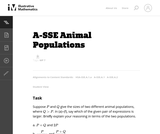Unrestricted Use
CC BY
Rating
0,0 stars

In this task students have to interpret expressions involving two variables in the context of a real world situation. All given expressions can be interpreted as quantities that one might study when looking at two animal populations.

نوع المادة:
Activity/Lab
Provider:
Illustrative Mathematics
Provider Set:
Illustrative Mathematics
المؤلف:
Illustrative Mathematics
05/01/2012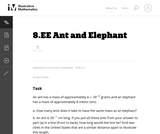Unrestricted Use
CC BY
Rating
0.0 stars

In this problem students are comparing a very small quantity with a very large quantity using the metric system. The metric system is especially convenient when comparing measurements using scientific notations since different units within the system are related by powers of ten.

Material Type:
Activity/Lab
Provider:
Illustrative Mathematics
Provider Set:
Illustrative Mathematics
Author:
Illustrative Mathematics
05/01/2012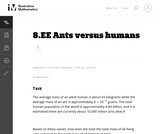Unrestricted Use
CC BY
Rating
0,0 stars

This task requires students to work with very large and small values expressed both in scientific notation and in decimal notation (standard form). In addition, students need to convert units of mass.

نوع المادة:
Activity/Lab
Provider:
Illustrative Mathematics
Provider Set:
Illustrative Mathematics
المؤلف:
Illustrative Mathematics
08/21/2012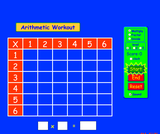Unrestricted Use
CC BY
Rating
5.0 stars

Brush up on your multiplication, division, and factoring skills with this interactive multiplication chart. Three levels and timed or untimed options are available.

Material Type:
Simulation
Provider:
Provider Set:
PhET Interactive Simulations
Author:
Michael Dubson
11/05/2011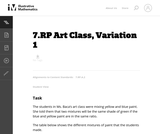Unrestricted Use
CC BY
Rating
0,0 stars

This this task about mixing paint requires students to graph ratios on a coordinate plane. It is a standard language in ratio problem.

نوع المادة:
Activity/Lab
Provider:
Illustrative Mathematics
Provider Set:
Illustrative Mathematics
المؤلف:
Illustrative Mathematics
05/01/2012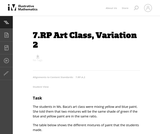Unrestricted Use
CC BY
Rating
0,0 stars

This this task about mixing paint requires students to graph ratios on a coordinate plane. It is a standard language in ratio problem.

نوع المادة:
Activity/Lab
Provider:
Illustrative Mathematics
Provider Set:
Illustrative Mathematics
المؤلف:
Illustrative Mathematics
05/01/2012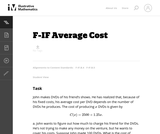Unrestricted Use
CC BY
Rating
0,0 stars

In this real world problem students solve questions based on the relationship between production costs and price.

نوع المادة:
Activity/Lab
Provider:
Illustrative Mathematics
Provider Set:
Illustrative Mathematics
المؤلف:
Illustrative Mathematics
05/01/2012Unrestricted Use
CC BY
Rating
0,0 stars

This task provides a real world context for interpreting and solving exponential equations. There are two solutions provided for part (a). The first solution demonstrates how to deduce the conclusion by thinking in terms of the functions and their rates of change. The second approach illustrates a rigorous algebraic demonstration that the two populations can never be equal.

نوع المادة:
Activity/Lab
Provider:
Illustrative Mathematics
Provider Set:
Illustrative Mathematics
المؤلف:
Illustrative Mathematics
05/01/2012Unrestricted Use
CC BY
Rating
0,0 stars

نوع المادة:
Activity/Lab
Provider:
Illustrative Mathematics
Provider Set:
Illustrative Mathematics
المؤلف:
Illustrative Mathematics
05/01/2012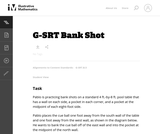Unrestricted Use
CC BY
Rating
0,0 stars

This task asks students to use similarity to solve a problem in a context that will be familiar to many, though most students are accustomed to using intuition rather than geometric reasoning to set up the shot.

نوع المادة:
Activity/Lab
Provider:
Illustrative Mathematics
Provider Set:
Illustrative Mathematics
المؤلف:
Illustrative Mathematics
08/21/2012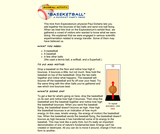Conditional Remix & Share Permitted
CC BY-NC-SA
Rating
0,0 stars

This trick from Exploratorium physicist Paul Doherty lets you add together the bounces of two balls and send one ball flying. When we tried this trick on the Exploratorium's exhibit floor, we gathered a crowd of visitors who wanted to know what we were doing. We explained that we were engaged in serious scientific experimentation related to energy transfer. Some of them may have believed us. If you'd like to go into the physical calculations of this phenomenam, see the related resource "Bouncing Balls" - it's the same activity but with the math explained.

نوع المادة:
Activity/Lab
Provider:
متحف Exploratorium لدعم التعليم
المؤلف:
Paul Doherty
The Exploratorium
11/07/2012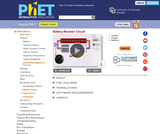Unrestricted Use
CC BY
Rating
0.0 stars

Look inside a resistor to see how it works. Increase the battery voltage to make more electrons flow though the resistor. Increase the resistance to block the flow of electrons. Watch the current and resistor temperature change.

Material Type:
Simulation
Provider:
Provider Set:
PhET Interactive Simulations
Author:
Carl Wieman
Sam Reid
11/20/2008Only Sharing Permitted
CC BY-NC-ND
Rating
0.0 stars

This activity explores the main algorithms that are used as the basis for searching on computers, using different variations on the game of battleships. This activity demonstrates three search methods for finding information in data: linear searching, binary searching and hashing. It also includes an optional introductory activity as well as a video showing a fun demonstration related to the same content.

Material Type:
Activity/Lab
Game
Lesson Plan
Simulation
Provider:
Author:
Brian Mason Scientific and Technical Trust
Computer Science Unplugged
Ian Witten
Jane McKenzie
Mike Fellows
Tim Bell
01/02/2002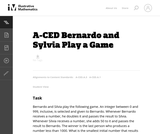Unrestricted Use
CC BY
Rating
0,0 stars

This task presents a simple but mathematically interesting game whose solution is a challenging exercise in creating and reasoning with algebraic inequalities. The core of the task involves converting a verbal statement into a mathematical inequality in a context in which the inequality is not obviously presented, and then repeatedly using the inequality to deduce information about the structure of the game.

نوع المادة:
Activity/Lab
Provider:
Illustrative Mathematics
Provider Set:
Illustrative Mathematics
المؤلف:
Illustrative Mathematics
10/21/2013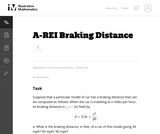Unrestricted Use
CC BY
Rating
0,0 stars

This task provides an exploration of a quadratic equation by descriptive, numerical, graphical, and algebraic techniques. Based on its real-world applicability, teachers could use the task as a way to introduce and motivate algebraic techniques like completing the square, en route to a derivation of the quadratic formula.

نوع المادة:
Activity/Lab
Provider:
Illustrative Mathematics
Provider Set:
Illustrative Mathematics
المؤلف:
Illustrative Mathematics
05/01/2012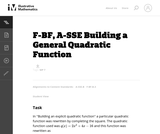Unrestricted Use
CC BY
Rating
0,0 stars

This task is for instructional purposes only and builds on ``Building an explicit quadratic function.''

نوع المادة:
Activity/Lab
Provider:
Illustrative Mathematics
Provider Set:
Illustrative Mathematics
المؤلف:
Illustrative Mathematics
08/20/2012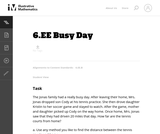Unrestricted Use
CC BY
Rating
3,0 stars

This task provides a good entry point for students into representing quantities in contexts with variables and expressions and building equations that reflect the relationships presented in the context.

نوع المادة:
Activity/Lab
Provider:
Illustrative Mathematics
Provider Set:
Illustrative Mathematics
المؤلف:
Illustrative Mathematics
08/15/2012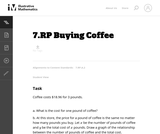Unrestricted Use
CC BY
Rating
0.0 stars

This is a task where it would be appropriate for students to use technology such as a graphing calculator or GeoGebra, making it a good candidate for students to engage in Standard for Mathematical Practice 5 Use appropriate tools strategically.

Material Type:
Activity/Lab
Provider:
Illustrative Mathematics
Provider Set:
Illustrative Mathematics
Author:
Illustrative Mathematics
05/01/2012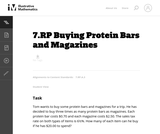Unrestricted Use
CC BY
Rating
0.0 stars

This task requires students to solves multistep ratio and percent problem.

Material Type:
Activity/Lab
Provider:
Illustrative Mathematics
Provider Set:
Illustrative Mathematics
Author:
Illustrative Mathematics
05/01/2012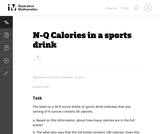Unrestricted Use
CC BY
Rating
0.0 stars

This problem involves the meaning of numbers found on labels. When the level of accuracy is not given we need to make assumptions based on how the information is reported. The goal of the task is to stimulate a conversation about rounding and about how to record numbers with an appropriate level of accuracy, tying in directly to the standard N-Q.3. It is therefore better suited for instruction than for assessment purposes.

Material Type:
Activity/Lab
Provider:
Illustrative Mathematics
Provider Set:
Illustrative Mathematics
Author:
Illustrative Mathematics
01/05/2013Unrestricted Use
CC BY
Rating
0.0 stars

This task presents a real-world problem requiring the students to write linear equations to model different cell phone plans. Looking at the graphs of the lines in the context of the cell phone plans allows the students to connect the meaning of the intersection points of two lines with the simultaneous solution of two linear equations.

Material Type:
Activity/Lab
Provider:
Illustrative Mathematics
Provider Set:
Illustrative Mathematics
Author:
Illustrative Mathematics
05/01/2012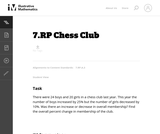Unrestricted Use
CC BY
Rating
0.0 stars

This problem includes a percent increase in one part with a percent decrease in the remaining and asks students to find the overall percent change. The problem may be solved using proportions or by reasoning through the computations or writing a set of equations.

Material Type:
Activity/Lab
Provider:
Illustrative Mathematics
Provider Set:
Illustrative Mathematics
Author:
Illustrative Mathematics
05/01/2012Unrestricted Use
CC BY
Rating
0.0 stars

This task presents a real world situation that can be modeled with a linear function best suited for an instructional context.

Material Type:
Activity/Lab
Provider:
Illustrative Mathematics
Provider Set:
Illustrative Mathematics
Author:
Illustrative Mathematics
05/01/2012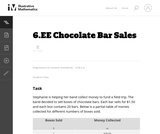Unrestricted Use
CC BY
Rating
0.0 stars

In this task students use different representations to analyze the relationship between two quantities and to solve a real world problem. The situation presented provides a good opportunity to make connections between the information provided by tables, graphs and equations.

Material Type:
Activity/Lab
Provider:
Illustrative Mathematics
Provider Set:
Illustrative Mathematics
Author:
Illustrative Mathematics
08/08/2012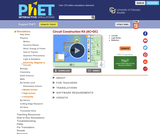Unrestricted Use
CC BY
Rating
0.0 stars

This new version of the CCK adds capacitors, inductors and AC voltage sources to your toolbox! Now you can graph the current and voltage as a function of time.

Material Type:
Simulation
Provider:
Provider Set:
PhET Interactive Simulations
Author:
Carl Wieman
Kathy Perkins
Michael Dubson
Sam Reid
07/12/2008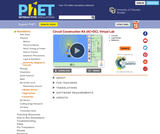Unrestricted Use
CC BY
Rating
0.0 stars

Build circuits with capacitors, inductors, resistors and AC or DC voltage sources, and inspect them using lab instruments such as voltmeters and ammeters.

Material Type:
Simulation
Provider:
Provider Set:
PhET Interactive Simulations
Author:
Carl Wieman
Kathy Perkins
Michael Dubson
Sam Reid
07/01/2006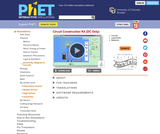Unrestricted Use
CC BY
Rating
0.0 stars

An electronics kit in your computer! Build circuits with resistors, light bulbs, batteries, and switches. Take measurements with the realistic ammeter and voltmeter. View the circuit as a schematic diagram, or switch to a life-like view.

Material Type:
Simulation
Provider:
Provider Set:
PhET Interactive Simulations
Author:
Carl Wieman
Kathy Perkins
Michael Dubson
Sam Reid
06/15/2005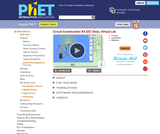Unrestricted Use
CC BY
Rating
4.0 stars

Build circuits with resistors, light bulbs, batteries, and switches and take measurements with laboratory equipment like the realistic ammeter and voltmeter.

Material Type:
Simulation
Provider:
Provider Set:
PhET Interactive Simulations
Author:
Carl Wieman
Kathy Perkins
Michael Dubson
Sam Reid
07/01/2004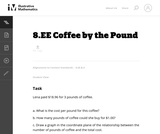Unrestricted Use
CC BY
Rating
0.0 stars

Students are exptected to identify the slope of the line with the unit rate in this real world problem.

Material Type:
Activity/Lab
Provider:
Illustrative Mathematics
Provider Set:
Illustrative Mathematics
Author:
Illustrative Mathematics
05/01/2012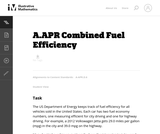Unrestricted Use
CC BY
Rating
0.0 stars

The primary purpose of this problem is to rewrite simple rational expressions in different forms to exhibit different aspects of the expression, in the context of a relevant real-world context (the fuel efficiency of of a car). Indeed, the given form of the combined fuel economy computation is useful for direct calculation, but if asked for an approximation, is not particularly helpful.

Material Type:
Activity/Lab
Provider:
Illustrative Mathematics
Provider Set:
Illustrative Mathematics
Author:
Illustrative Mathematics
01/25/2013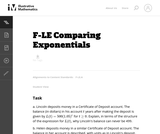Unrestricted Use
CC BY
Rating
0.0 stars

This task gives students an opportunity to work with exponential functions in a real world context involving continuously compounded interest. They will study how the base of the exponential function impacts its growth rate and use logarithms to solve exponential equations.

Material Type:
Activity/Lab
Provider:
Illustrative Mathematics
Provider Set:
Illustrative Mathematics
Author:
Illustrative Mathematics
05/01/2012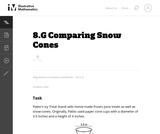Unrestricted Use
CC BY
Rating
0.0 stars

Students must calculate the volume of a cone in this real world task.

Material Type:
Activity/Lab
Provider:
Illustrative Mathematics
Provider Set:
Illustrative Mathematics
Author:
Illustrative Mathematics
05/01/2012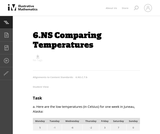Unrestricted Use
CC BY
Rating
0.0 stars

The purpose of the task is for students to compare signed numbers in a real-world context.

Material Type:
Activity/Lab
Provider:
Illustrative Mathematics
Provider Set:
Illustrative Mathematics
Author:
Illustrative Mathematics
07/23/2012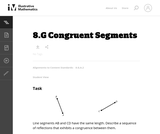Unrestricted Use
CC BY
Rating
0.0 stars

Students' first experience with transformations is likely to be with specific shapes like triangles, quadrilaterals, circles, and figures with symmetry. Exhibiting a sequence of transformations that shows that two generic line segments of the same length are congruent is a good way for students to begin thinking about transformations in greater generality.

Material Type:
Activity/Lab
Provider:
Illustrative Mathematics
Provider Set:
Illustrative Mathematics
Author:
Illustrative Mathematics
05/01/2012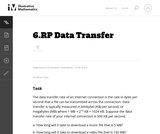Unrestricted Use
CC BY
Rating
0.0 stars

This task asks the students to solve a real-world problem involving unit rates (data per unit time) using units that many teens and pre-teens have heard of but may not know the definition for. While the computations involved are not particularly complex, the units will be abstract for many students.

Material Type:
Activity/Lab
Provider:
Illustrative Mathematics
Provider Set:
Illustrative Mathematics
Author:
Illustrative Mathematics
12/14/2012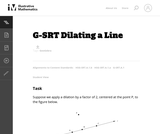Unrestricted Use
CC BY
Rating
0.0 stars

This task gives students the opportunity to verify that a dilation takes a line that does not pass through the center to a line parallel to the original line, and to verify that a dilation of a line segment (whether it passes through the center or not) is longer or shorter by the scale factor.

Material Type:
Activity/Lab
Provider:
Illustrative Mathematics
Provider Set:
Illustrative Mathematics
Author:
Illustrative Mathematics
05/01/2012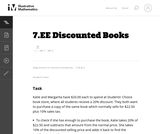Unrestricted Use
CC BY
Rating
0.0 stars

This purpose of this task is to help students see two different ways to look at percentages both as a decrease and an increase of an original amount. In addition, students have to turn a verbal description of several operations into mathematical symbols.

Material Type:
Activity/Lab
Provider:
Illustrative Mathematics
Provider Set:
Illustrative Mathematics
Author:
Illustrative Mathematics